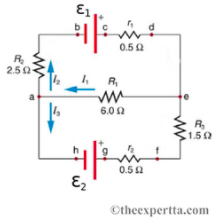# Problem: Consider the following circuit of three resistors (R1, R2, and R3), with batteries that have emfs ε1 = 11 V and ε2 = 44.5 V, and internal resistances r1 and r2.Part A. Find the current I1, in amps.Part B. Find the current I2, in amps.Part C. find the current I3, in amps.

###### FREE Expert Solution

Kirchhoff's voltage law:

$\overline{){\mathbf{\Sigma }}{\mathbf{V}}{\mathbf{=}}{\mathbf{0}}}$

Voltage:

$\overline{){\mathbf{V}}{\mathbf{=}}{\mathbf{i}}{\mathbf{R}}}$

Let's apply Kirchhoff's loop rule to the top closed loop in a clockwise direction:

$\begin{array}{rcl}{\mathbf{\epsilon }}_{\mathbf{1}}\mathbf{-}{\mathbf{I}}_{\mathbf{2}}{\mathbf{r}}_{\mathbf{1}}\mathbf{-}{\mathbf{I}}_{\mathbf{1}}{\mathbf{R}}_{\mathbf{1}}\mathbf{-}{\mathbf{I}}_{\mathbf{2}}{\mathbf{R}}_{\mathbf{2}}& \mathbf{=}& \mathbf{0}\\ \mathbf{11}\mathbf{-}\mathbf{0}\mathbf{.}\mathbf{5}{\mathbf{I}}_{\mathbf{2}}\mathbf{-}\mathbf{6}\mathbf{.}\mathbf{0}{\mathbf{I}}_{\mathbf{1}}\mathbf{-}\mathbf{2}\mathbf{.}\mathbf{5}{\mathbf{I}}_{\mathbf{2}}& \mathbf{=}& \mathbf{0}\\ \mathbf{11}\mathbf{-}\mathbf{6}\mathbf{.}\mathbf{0}{\mathbf{I}}_{\mathbf{1}}\mathbf{-}\mathbf{3}\mathbf{.}\mathbf{0}{\mathbf{I}}_{\mathbf{2}}& \mathbf{=}& \mathbf{0}\end{array}$

6.0I1 + 3.0I2 = 11 ...................... (1)

100% (115 ratings)###### Problem Details

Consider the following circuit of three resistors (R1, R2, and R3), with batteries that have emfs ε1 = 11 V and ε2 = 44.5 V, and internal resistances r1 and r2.Part A. Find the current I1, in amps.

Part B. Find the current I2, in amps.

Part C. find the current I3, in amps.